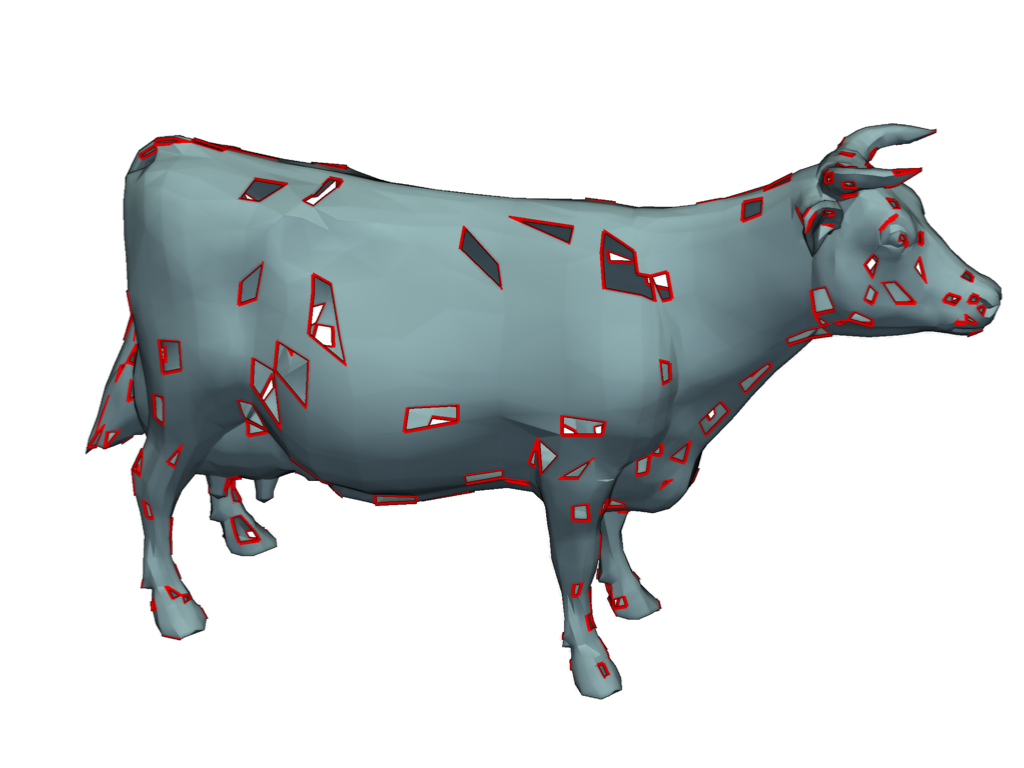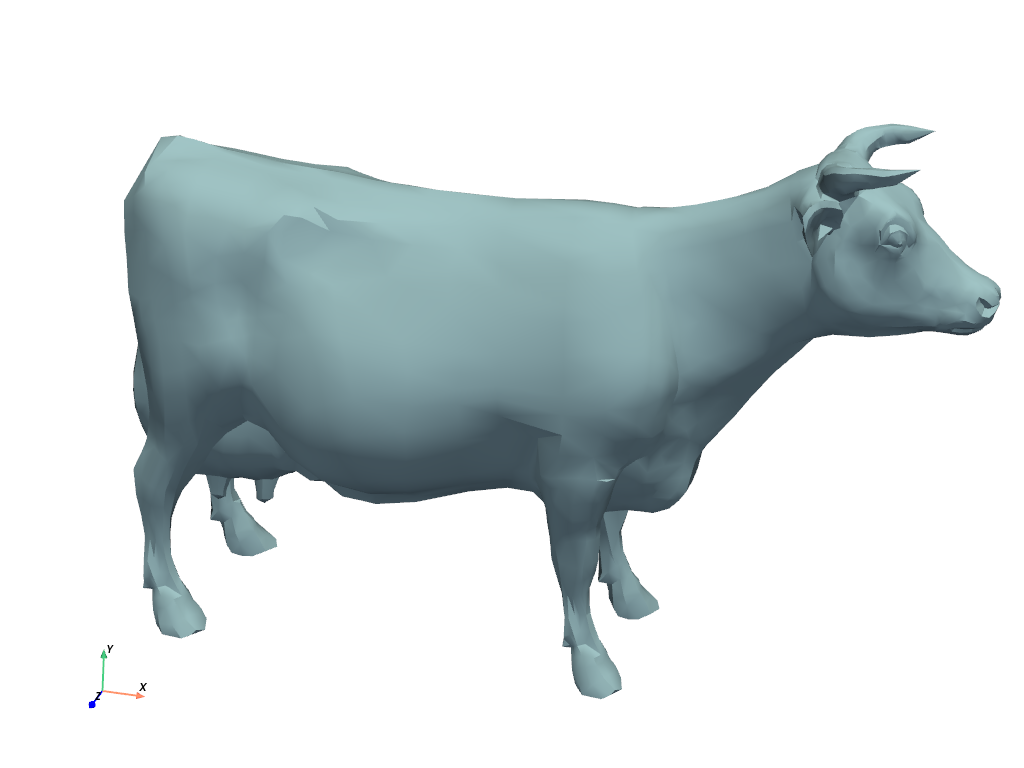# Holey Cow#

Repair a holey cow

```import numpy as np
import pymeshfix as mf

# sphinx_gallery_thumbnail_number = 1
import pyvista as pv
from pyvista import examples
```
```cow = examples.download_cow()

# Add holes and cast to triangulated PolyData
cow["random"] = np.random.rand(cow.n_cells)
holy_cow = cow.threshold(0.9, invert=True).extract_geometry().triangulate()
print(holy_cow)
```
```PolyData (0x7f0cb5e57d60)
N Cells:      5214
N Points:     2903
N Strips:     0
X Bounds:     -4.446e+00, 5.998e+00
Y Bounds:     -3.637e+00, 2.760e+00
Z Bounds:     -1.701e+00, 1.701e+00
N Arrays:     1
```
```# A nice camera location of the cow
cpos = [(6.56, 8.73, 22.03), (0.77, -0.44, 0.0), (-0.13, 0.93, -0.35)]

meshfix = mf.MeshFix(holy_cow)
holes = meshfix.extract_holes()

# Render the mesh and outline the holes
p = pv.Plotter()
p.camera_position = cpos
p.enable_eye_dome_lighting()  # helps depth perception
p.show()
```Repair the holey cow

```meshfix.repair(verbose=True)
```
```Patching holes...
Patched 210 holes
Fixing degeneracies and intersections
```

Show the repaired result

```repaired = meshfix.mesh
print(repaired)
```
```PolyData (0x7f0cb5c7fe20)
N Cells:      5698
N Points:     2851
N Strips:     0
X Bounds:     -4.446e+00, 5.998e+00
Y Bounds:     -3.637e+00, 2.760e+00
Z Bounds:     -1.701e+00, 1.701e+00
N Arrays:     0
```
```repaired.plot(cpos=cpos)
```Total running time of the script: ( 0 minutes 1.696 seconds)

Gallery generated by Sphinx-Gallery